#### The 2D case

The dynamics of a 2D rigid body that moves in the plane can be handled as a special case of a 3D body. Let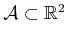be a 2D body, expressed in its body frame. The total external forces acting on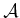can be expressed in terms of a two-dimensional total force through the center of mass and a moment through the center of mass. The phase space for this model has six dimensions. Three come from the degrees of freedom of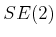, two come from linear velocity, and one comes from angular velocity.

The translational part is once again expressed as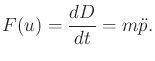(13.107)

This provides four components of the state transition equation.

All rotations must occur with respect to the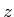-axis in the 2D formulation. This means that the angular velocity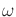is a scalar value. Let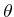denote the orientation of. The relationship betweenandis given by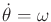, which yields one more component of the state transition equation.

At this point, only one component remains. Recall (13.92). By inspecting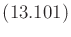it can be seen that the inertia-based terms vanish. In that formulation,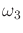is equivalent to the scalarfor the 2D case. The final terms of all three equations vanish because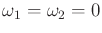. The first terms of the first two equations also vanish because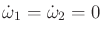. This leaves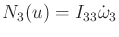. In the 2D case, this can be notationally simplified to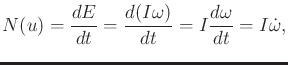(13.108)

in which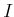is now a scalar. Note that for the 3D case, the angular velocity can change, even when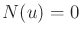. In the 2D case, however, this is not possible. In both cases, the moment of momentum is conserved; in the 2D case, this happens to imply thatis fixed. The sixth component of the state transition equation is obtained by solving (13.108) for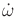.

The state transition equation for a 2D rigid body in the plane is therefore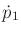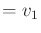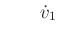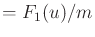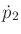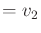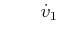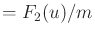(13.109)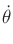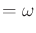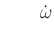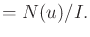Steven M LaValle 2020-08-14